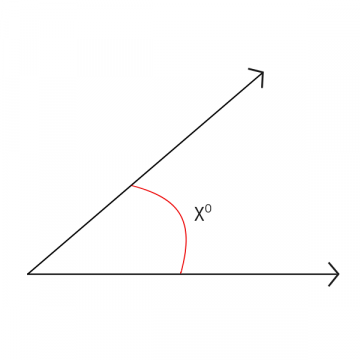# Finding Angles | Different Systems Used to Measure Angles

Go back to  'Math Concepts'

### July 13, 2020

Geometry creates space for students to draw and learn 2D and 3D shapes. But where does it begin? Lines and angles- the first concepts of geometry that a student studies. A line is set of points, while an angle is made when two rays intersect. A ray is a line, which extends infinitely in one direction.

When two rays originate from a common point, the inclination of one ray on another is called an angle.### Why are Angles Important?

The uses of angles are vast ranging from fun uses like in a game of carrom to more complex ones like planetary motion.

Construction, architecture, sports, engineering, art, dance etc. make use of the concept of angles. That apart, scientists and astronomers depend on the angles that celestial bodies make to study their movement and get down to concrete conclusions.

## Standard Systems Used to Measure Angles

Here are the various standard systems used to measure angles. We have simplified them for you to help your students

### 1. Degree

A degree is a measurement of a plane angle, representing 1/360 of a full rotation. It is usually denoted with °.

Why is 360° considered as the angle made for one complete rotation?

It is believed that the Babylonians invented the degree and always counted in base-60. This is where the initial ideas of 360 came about. It was considered due to it’s readily divisible nature by many numbers. For example, 360 is divisible by every number between 1 to 10 except 7. Science also confirms that the Earth takes close to 365 days to complete one revolution around the sun. Most major calendars have close to 360 days in a year.

Degrees are measured using protractors and is one of the most common methods of measuring angles.Radian measure of an angle is defined as the ratio of the length of the arc that the angle subtends of a circle, divided by the length of the radius of the same circle.

This ratio will give you the radian measure of the angle.A radian does not have a unit. Why? Because it is the ratio of two lengths, hence the units get cancelled.

There are 2? radians in a full circle and 1 radian is approximately equal to 57° as shown below.### 3. Revolution

A revolution is said to be the simplest, most natural unit of measure for geometric angles. Revolutions help students understand the deeper meaning of angles. This is because an angle is basically a subdivision of a circle rather than the sum of a few degrees. For example, it’s easier for students to understand, “a right angle is quarter of a circle” than, “a right angle is 90°

Introducing your students to revolutions alongside degrees will help them grasp the concepts of angles in a much more stronger manner. Here’s an illustration which you can use to explain the difference between the three systems of measuring anglesApart from the standard systems, it is good to build the idea early on in the student that the unit to measure an angle is an angle. It does not “have” to be measured using degrees. They can create their own system of measurement and use that to measure angles.

It is easier for students to develop the sense of measuring an angle using a wedge rather than a protractor. A paper wedge protractor can be made by folding a circle in halves four times until 16 wedges (or sectors) have been formed.If the paper is see through then the paper can be placed over a corner of a polygon or an angle ray. The number of wedges can be counted.

Encourage your students to discover new methods of measuring angles and who knows, in the years to come, their method may become the conventional method.

Cuemath, being one of the leading platforms to provide a dynamic classroom-like environment at homes, believes in active learning and participation. Therefore, we duly come up with updates that will help our student base to develop continuous learning. Cuemath has always provided its students with unconditional support to help them achieve their academic goals and will continue providing the same.  With Cuemath's Live Online Classes you will get a 100% personalized course, instant doubt clearing, and India's best math teachers. We also have a maths gym app that will help your kids to develop mental skills. This app is available for both iOS and Android platforms. Come, join Cuemath for a bright future.

0